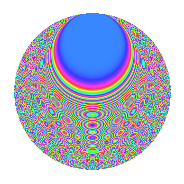# Properties

 Label 3150.2.uLevel 3150 Weight 2 Character orbit u Rep. character $$\chi_{3150}(551,\cdot)$$ Character field $$\Q(\zeta_{6})$$ Dimension 304 Sturm bound 1440

# Related objects

## Defining parameters

 Level: $$N$$ = $$3150 = 2 \cdot 3^{2} \cdot 5^{2} \cdot 7$$ Weight: $$k$$ = $$2$$ Character orbit: $$[\chi]$$ = 3150.u (of order $$6$$ and degree $$2$$) Character conductor: $$\operatorname{cond}(\chi)$$ = $$63$$ Character field: $$\Q(\zeta_{6})$$ Sturm bound: $$1440$$

## Dimensions

The following table gives the dimensions of various subspaces of $$M_{2}(3150, [\chi])$$.

Total New Old
Modular forms 1488 304 1184
Cusp forms 1392 304 1088
Eisenstein series 96 0 96

## Trace form

 $$304q + 152q^{4} + 2q^{7} + 6q^{9} + O(q^{10})$$ $$304q + 152q^{4} + 2q^{7} + 6q^{9} - 6q^{13} + 6q^{14} - 152q^{16} - 18q^{17} - 4q^{18} - 2q^{21} + 6q^{24} - 12q^{26} - 2q^{28} - 6q^{29} + 6q^{31} - 42q^{33} - 2q^{37} + 6q^{39} - 6q^{41} + 2q^{42} - 2q^{43} - 12q^{44} - 6q^{46} - 18q^{47} - 14q^{49} - 4q^{51} + 36q^{53} + 18q^{54} + 6q^{57} + 12q^{58} + 30q^{59} - 24q^{61} + 36q^{62} + 38q^{63} - 304q^{64} + 14q^{67} - 36q^{68} - 42q^{69} - 8q^{72} + 30q^{77} - 16q^{78} + 20q^{79} - 14q^{81} + 2q^{84} - 48q^{87} - 24q^{89} - 12q^{91} + 30q^{92} - 38q^{93} + 6q^{96} - 6q^{97} + 24q^{98} + 10q^{99} + O(q^{100})$$

## Decomposition of $$S_{2}^{\mathrm{new}}(3150, [\chi])$$ into newform subspaces

The newforms in this space have not yet been added to the LMFDB.

## Decomposition of $$S_{2}^{\mathrm{old}}(3150, [\chi])$$ into lower level spaces

$$S_{2}^{\mathrm{old}}(3150, [\chi]) \cong$$ $$S_{2}^{\mathrm{new}}(63, [\chi])$$$$^{\oplus 6}$$$$\oplus$$$$S_{2}^{\mathrm{new}}(126, [\chi])$$$$^{\oplus 3}$$$$\oplus$$$$S_{2}^{\mathrm{new}}(315, [\chi])$$$$^{\oplus 4}$$$$\oplus$$$$S_{2}^{\mathrm{new}}(630, [\chi])$$$$^{\oplus 2}$$$$\oplus$$$$S_{2}^{\mathrm{new}}(1575, [\chi])$$$$^{\oplus 2}$$

## Hecke Characteristic Polynomials

There are no characteristic polynomials of Hecke operators in the database